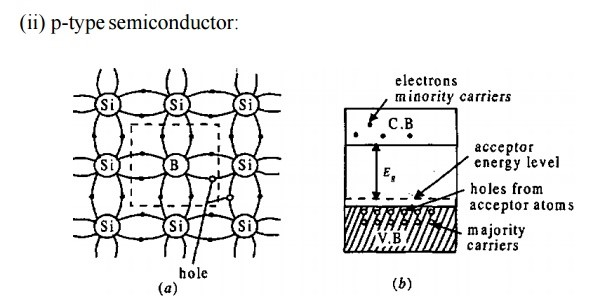# Classification of Semiconductor

Classification of Semiconductor

• Intrinsic Semiconductor
• Extrinsic Semiconductor

## Intrinsic Semiconductor

An intrinsic semiconductor also called an undoped semiconductor or i- type semiconductor.

It is a pure semiconductor without any significant dopant species present.

The number of charge carriers determined by the properties of the m aterial itself instead of the amount of impurities.

In intrinsic semiconductors the number of excited electrons and the number of holes are equal: n = p.

### Conductivity of Intrinsic semiconductor

• The electrical conductivity of intrinsic semiconductors can be due to crystal defects or to thermal excitation.
• Both electrons and holes contribute to current flow in an intrinsic semiconductor.
• The current which will flow in an intrinsic semiconductor consists of both electron and hole current.
• That is, the electrons which have been freed from their lattice positions into the conduction band can move through the material.
• In addition, other electrons can hop between lattice positions to fill the vacancies left by the freed electrons.
• This additional mechanism is called hole conduction because it is as if the  holes  are migrating across the material in the direction opposite to the free electron movement.
• The current flow in an intrinsic semiconductor is influenced by the density of energy states which in turn influences the electron density in the conduction band.
• This current is highly temperature dependent.

#### Thermal excitation:

In an intrinsic semiconductor like silicon at temperatures above absolute zero, there will be some electrons which are excited across the band gap into the conduction band and which can produce current.

When the electron in pure silicon crosses the gap, it leaves behind an electron vacancy or “hole” in the regular silicon lattice.

Under the influence of an external voltage, both the electron and the hole can move across the material.

##### In n-type semiconductor:

The dopant contributes extra electrons, dramatically increasing the conductivity

##### In p-type semiconductor:

The dopant produces extra vacancies or holes, which likewise increase the conductivity.

## Extrinsic Semiconductor

• The electrical conductivity of a pure semiconductor is very small.
• To increase the conductivity, impurities are added.
• The impurity added semiconductor is called extrinsic semiconductor.
• The process of adding impurity is called doping.
• The added impurity is called dopant.
• Usually one or two atoms of impurity is added per 106 atoms of a semiconductor.
• There are two types (i) p-type and (ii) n-type semiconductors.
• When an impurity, from V group elements like arsenic (As), antimony having 5 valence electrons is added to Ge (or Si), the impurity atom donates one electron to Ge (or Si).
• The 4 electrons of the impurity atom is engaged in covalent bonding with Si atom.
• The fifth electron is free. This increases the conductivity.
• The impurities are called donors.
• The impurity added semiconductor is called n-type semiconductor, because their increased conductivity is due to the presence of the negatively charged electrons, which are called the majority carriers.
• The energy band of the electrons donated by the impurity atoms is just below the conduction band.
• The electrons absorb thermal energy and occupy the conduction band.
• Due to the breaking of covalent bond, there will be a few holes in the valence band at this temperature.
• These holes in n-type are called minority carriers.• If a III group element, like indium (In), boron (B), aluminium (AI) etc., having three valence electrons, is added to a semiconductor say Si, the three electrons form covalent bond.
• There is a deficiency of one electron to complete the 4th covalent bond and is called a hole.The presence of the hole increases the conductivity because these holes move to the nearby atom, at the same time the electrons move in the opposite direction.
• The impurities added semiconductor is called p-type semiconductor.
• The impurities are called acceptors as they accept electrons from the semiconductor
• Holes are the majority carriers and the electrons produced by the breaking of bonds are the minority carriers.

To see other topics in Basic Electrical and Electronics Engineering Click here

Construction of DC GENERATOR

Semiconductor Devices And Applications

Classification of Materials

PN Junction Diode

Zener Effects

Rectifiers and Types of rectifiers

Bipolar Junction Transistor(BJT)